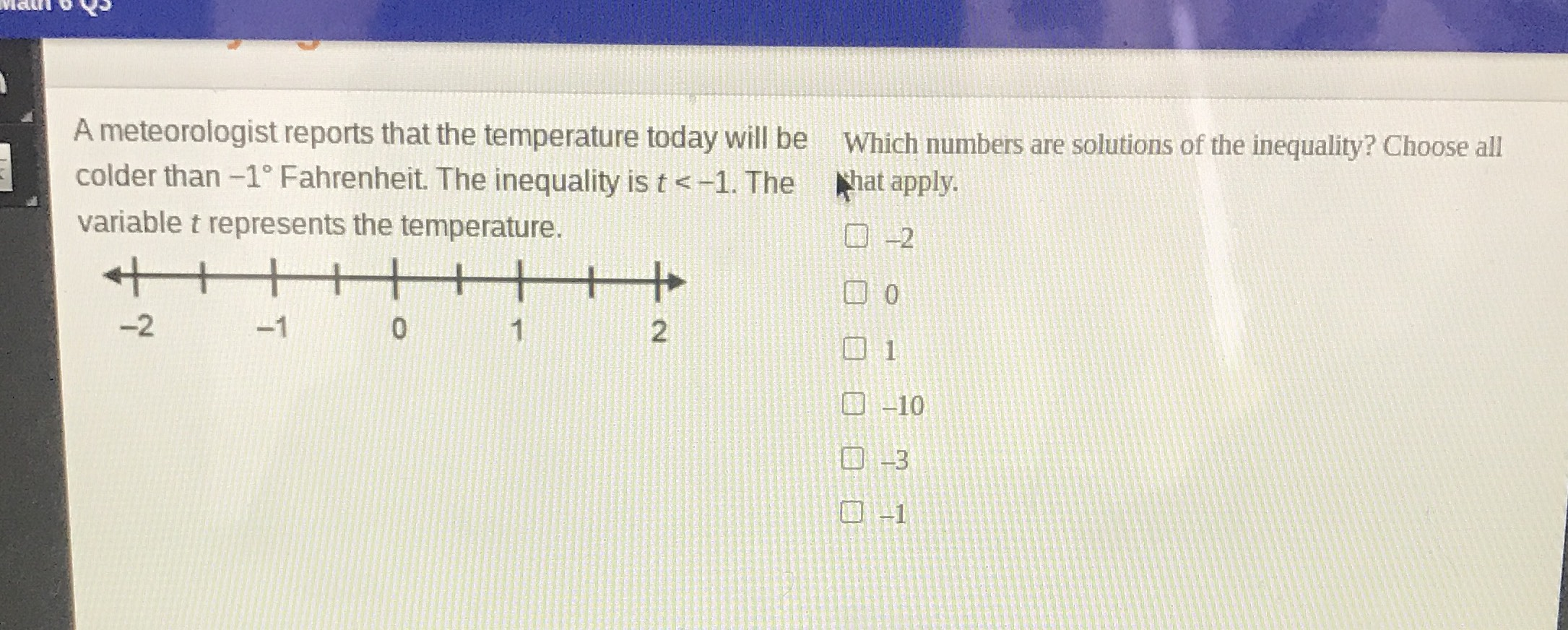### ¿Todavía tienes preguntas de matemáticas?

Pregunte a nuestros tutores expertos
Algebra
PreguntaA meteorologist reports that the temperature today will be colder than $$- 1 ^ { \circ }$$ Fahrenheit. The inequality is $$t < - 1$$ . The variable $$t$$ represents the temperature. Which numbers are solutions of the inequality? Choose all That apply. $$- 2$$

$$0$$

$$1$$

$$- 10$$

$$- 3$$

$$- 1$$

$$- 2,- 10,- 3$$